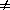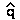The Mathematical Theory of Natural Selection

Natural selection is the name given to an evolutionary process.

If:     variation exists for some trait, and
a fitness difference is correlated with that trait, and
the trait is to some degree heritable (determined by genetics),
Then: the trait distribution will change
over the life history of organisms in a single generation, and
between generations.

The process of change is called "adaptation".

Or, "Natural Selection" describes a process in which
"adaptation" occurs in such a way that "fitness" increases.
Under certain conditions, this results in descent with modification.

The General Selection Model

Evolution & Natural Selection can be modeled genetically.

Natural Selection results in change of allele frequency (q) [read as "delta q"]
in consequence of differences in the relative fitness (W)
of the phenotypes to which the alleles contribute.

Fitness is a phenotype of individual organisms.
Fitness is determined genetically (at least in part).
Fitness is related to success at survival AND reproduction.
Fitness can be measured & quantified (see below).
i.e., the relative fitness of genotypes can be assigned numerical values.

The consequences of natural selection depend on the dominance of fitness:
e.g., whether the "fit" phenotype is due to a dominant or recessive allele.

Then, allele frequency change is predicted by the General Selection Equation:q = [pq] [(q)(W2 - W1) + (p)(W1 - W0)] /where W0, W1, & W2 are the fitness phenotypes
of the AA, AB, & BB genotypes, respectively  [see derivation]

Consider the simplest case:  Complete Dominance

genotype:   AA     AB      BB
phenotype: W0WW2    (AA and AB have identical phenotypes)

Then the GSE simplifies toq = pq2(W2 - W1)     (since W1 - W0 = 0)

If 'B' phenotype is more fit than 'A' phenotype,
W2 > W1   &q > 0    so   q increases.

If 'B' phenotype is less fit than 'A' phenotype,
W2 < W1    &q < 0    so   q decreases.

then(W2 - W1) : the greater the difference in fitness,
the greater the intensity of selection
and the more rapid the change

A numerical example of Selection:
Tay-Sachs Disease is caused by an allele
that is rare         (q0.001)
recessive (W0 = W1 = 1)
lethal         (W2 = 0)

Thenq = pq2(W2 - W1) = -pq-q2   (since p1)

That is, Natural Selection results in a decrease in the frequency of
the Tay-Sachs allele of about one part in a million (0.0012) per generation

An alternate notation with selection coefficients simplifies the math

s = 1 - W

The selection coefficient (s) is the difference in fitness
of the phenotype relative to some 'standard' phenotype
that has a fitness  W = 1
[The math is simpler because only one variable is used for fitness.]

(1) Complete dominance

genotype:   AA      AB     BB
phenotype:  W0 =  WW2    (AA and AB have identical phenotypes)
or       1    =   11 - s

if  0 < s < 1 : 'B' is deleterious(at a selective disadvantage)
if  s < 0       : 'B' is advantageous

thenq = -spq2 / (1 - sq2)      [see derivation]

(2) Incomplete dominance

genotype:    AA     AB        BB
phenotype:  WWW2    (all phenotypes different)
or            1 - s11 - s2

if 0 < s1 & s2 < 1 : overdominance of fitness (heterozygote advantage)
The population has optimal fitness when both alleles are retained:
q will reach an equilibrium whereq = 0
0 << 1   (read as, "q hat")

then= (s1) / (s1 + s2)          [see derivation]

The General Selection Model: Summary

Direction of allele frequency change is due to fitness difference of alleles
(whether the effect of the allele on phenotype is deleterious or advantageous).
Ultimate consequences depend on the dominance of fitness
(whether the allele is dominant, semi-dominant, or recessive).
Rate of change is an interplay of both of these factors (see Lab #1)

AA   AB     BB    Consequence of natural selection   [ letq = change in f(B) ]

W0 =  W1 =  W2    No selection (neither allele has a selective advantage):
thenq = 0,  H-W proportions remain constant

W0 =  W1 >  W2    deleterious recessive (advantageous dominant):
thenq < 0,     q0.00  (loss): how fast? [Does it get there?]

W0 =  W1 <  W2    advantageous recessive (deleterious dominant):
thenq > 0,     q1.00  (fixation): how fast?

W0 <  W1 >  W2    overdominance [special case of semi-dominance]:
heterozygote superiority
q, whereq = 0

Text material © 2010 by Steven M. Carr